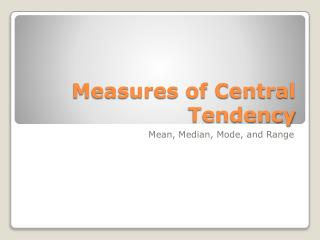DownloadDownload PresentationMeasures of Central Tendency

# Measures of Central Tendency

Télécharger la présentation## Measures of Central Tendency

- - - - - - - - - - - - - - - - - - - - - - - - - - - E N D - - - - - - - - - - - - - - - - - - - - - - - - - - -
##### Presentation Transcript

1. Measures of Central Tendency Mean, Median, Mode, and Range

2. Definitions- • Mean is the sum of all the data divided by the total number of observations. This is what we usually call the average. • Median is the central number when all the data is arranged in numerical order. • Mode is the data item appearing most often. There can be more than one or none. • Range is the difference between the smallest and largest number in the data set. Mean, Median, Mode, and Range

3. Given the list of test grades, find the mean: • 63, 77, 93, 63, 93, 90, 85, 81, 93, 89, 85, 101, 69 • To find the mean, add up all of the numbers and then divide by the total number given. • 63+77+93+63+93+90+85+81+93+89+85+ 101+69 = 1082 • 1082 ÷ 13 = 83.2 Mean

4. Given the list of test grades, find the median: • 63, 77, 93, 63, 93, 90, 85, 81, 93, 89, 85, 101, 69 • Put the grades in order. • 63, 63, 69, 77, 81, 85, 85, 89, 90, 93, 93, 93, 101 • What number is in the middle? • 85 Median

5. Given the list of test grades, find the mode: • 63, 77, 93, 63, 93, 90, 85, 81, 93, 89, 85, 101, 69 • What numbers are repeated? • 63 – 2 • 85 – 2 • 93 – 3 • Mode is 93. Mode

6. Given the list of test grades, find the range: • 63, 77, 93, 63, 93, 90, 85, 81, 93, 89, 85, 101, 69 • The biggest number is 101. • The smallest number is 63. • 101 – 63 = 38 Range

7. Turn to a partner and tell each other the definitions of each word: • Mean • Median • Mode • Range • I will give you 2 minutes! Mean, Median, Mode, and Range

8. Try one on your own: • Given the grams of protein per serving of some foods, find the mean, median, mode, and range: • 24, 35, 42, 10, 32, 24, 10, 18, 16, 18, 12, 30 • How did you do??? • Mean is about 22. • Median is 21. • Mode is 10, 18, 24. • Range is 32. Mean, Median, Mode, and Range